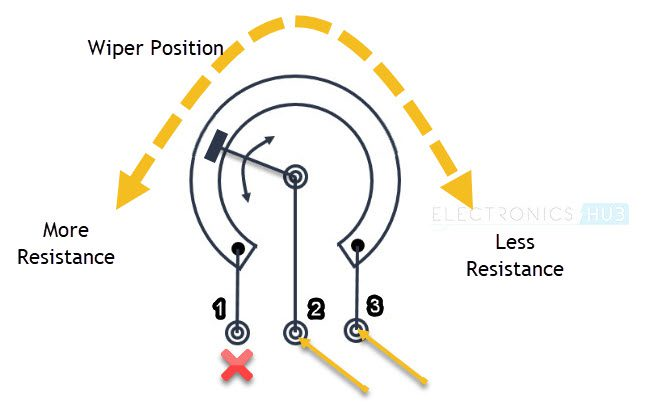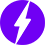# How a Potentiometer Works? Basics, Symbol, Types

In this tutorial, we will learn about Potentiometers. We will see what is a potentiometer, its construction and symbol, its working, different types of potentiometers, application of potentiometer as Rheostat and Voltage Divider and also Potentiometer Taper.

## What is a Potentiometer?

A Potentiometer or simply known as POT, is a three-terminal device with manually adjustable resistance using a movable contact. We all are familiar with Resistors. They are simple electrical devices which resist the flow of current in a circuit.

Normal resistors usually have fixed resistance. But what if you want vary the resistance as per the needs of your application? This is where Potentiometers come into play.

### Symbol and Construction

Since they are essentially resistors but with variable resistance, the symbol of a potentiometer is also close to that of a resistor. The following image shows the symbol of a potentiometer.

This symbol means a resistor with terminals 1 and 3 and sliding contact terminal 2. You will better understand this if we take a look at the construction of a typical potentiometer.

In rotating contact type potentiometers, there is a resistive track which is connected between terminals 1 and 3. A sliding contact, also known as Wiper, will move from end-to-end of the resistive track to vary the resistance.The external contacts are sometimes known as lugs.

## How Does a Potentiometer Work?

Let us now see how a potentiometer works. If you measure the resistance between the outer terminals, then you will always measure the maximum value (rated value) of that POT. For example, in case of a 10 KΩ POT, you will read full 10 KΩ between the outer terminals.

But, if you measure the resistance between one of the outer terminals and the Wiper terminal, then you will get a value that is dependent on the position of the Wiper. So, it can range from 0 KΩ to 10 KΩ in case of a 10 KΩ pot.

So, based on the terminals used, you can use the potentiometer as a simple Variable Resistor, also known as a Rheostat or a Voltage Divider.

### As Rheostat

A Potentiometer can work as a Rheostat by making use of just two of its terminals: One is the Wiper and the other is either of the two outer terminals. In this case, the POT acts as a two terminal variable resistor.

The value of the resistance increases or decreases by turning the Wiper.### As Voltage Divider

Another useful application of Potentiometers is they can be used as Voltage Dividers. If you remember, a Voltage Divider Circuit consists of two resistors connected in series. A voltage is applied at ends of the resistors and an output voltage is taken across a resistor.

This simple circuit is used to convert a high voltage to a low voltage. With the help of potentiometer, you can build a similar voltage divider by using all the three terminals.An input voltage is applied between the outer two terminals of the POT and the output voltage is taken between the wiper and the outer terminal which is connected to GND of the input supply.

Since a POT allows you to vary the resistance, you can essentially vary the output voltage of the Voltage Divider. Hence, this is a Voltage Divider with a variable output voltage.

## Different Types of Potentiometers

Even though the function is same, there are different types of Potentiometers available for various applications and use cases. Some of the popular types of POTs are mentioned below:

• Rotary POT
• Single Turn POT
• Multi Turn POT
• Linear POT
• Dual Gang POT
• Servo POT
• Trimmer POT
• Concentric POT
• Preset POT
• Digital POT

## Potentiometer Taper

There are several characteristics of Potentiometers but one that stands out is called the Taper. This characteristic is the relation between the position of the Wiper and the amount of resistance.

Potentiometers are usually classified as in to two types based on the Taper characteristic. They are:

• Linear Taper
• Logarithmic Taper### Linear Taper

The most commonly found Potentiometers are of Linear Tapper type. In this type, the relation between the position of the Wiper and the resistance is linear. This type of POTs are usually marked with letter ‘B’.### Logarithmic Taper

Another common but very application specific POT is the Logarithmic Taper or Log Taper. They are also called as Audio Taper as they are essentially used in audio control applications (volume control).

As the name suggests, in a log taper POT, the relation between resistance and wiper position is logarithmic. There are also Inverse Log Taper POTs. Log Taper POTs are usually marked with letter ‘A’.### Conclusion

A simple beginner’s tutorial on Potentiometers is presented here. You learned about some basics of Potentiometers, its working, electrical symbol and mechanical construction, two of the common applications and also different types.

We will be happy to hear your thoughts